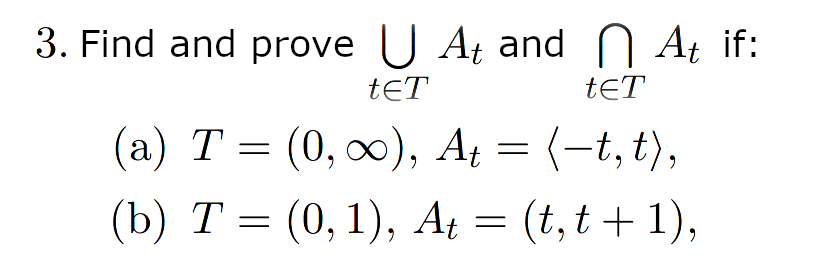# Proof of a set union and intersection

• MHB
bargaj
Hello!
Lately, I've been struggling with this assignment. (angle brackets represent closed interval)I figured out that:

a)
union = R
intersection = {0}
b)
union = (0, 2)
intersection = {1}

I asked my prof about this and she explained to me that it should be shown that if a set is an intersection of sets, then it belongs to each of those sets and, conversely, nothing else belongs to the intersection, so every other element does not belong to at least one of those sets. But I don't really know how to interpret this or where to even start. (normally, when proving the equality of two sets, I would try to prove that A⊆B and B⊆A, but I don't see how that's applicable here).

Gold Member
MHB
angle brackets represent closed interval
Never seen this notation. Usually closed intervals are denoted by square brackets.

a)
union = R
intersection = {0}
b)
union = (0, 2)
intersection = {1}
I agree.

I asked my prof about this and she explained to me that it should be shown that if a set is an intersection of sets, then it belongs to each of those sets and, conversely, nothing else belongs to the intersection, so every other element does not belong to at least one of those sets.
So we reached a consensus, right?

But I don't really know how to interpret this or where to even start.
You not only started, but also finished solving these problems.The professor just described the definition of intersection, which you have successfully applied, so you must have understood it.